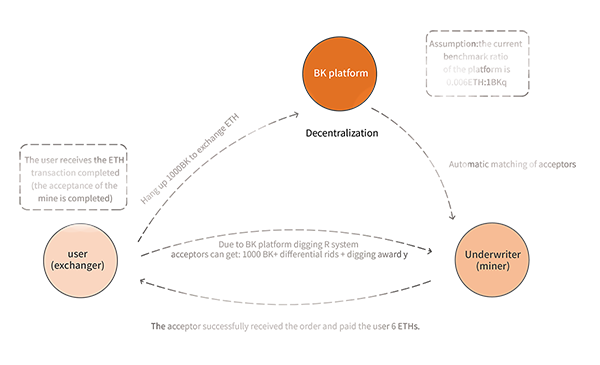### Acceptor mining award rules

It can be seen from the mining process that after the acceptor (miner) successfully realizes the currency exchange for the user, it means that the mining operation is completed, and the acceptor can obtain the platform mining reward. Namely: XXX BK+ difference subsidy + mining award, for a clearer definition of the mining reward rules, assuming that the user hangs out 1000BK wants to exchange ETH, the process is as follows:Spread subsidy: As the current market price of ETH is assumed to be 200 US dollars, the acceptor A is equivalent to paying 200*6=1200 USD. Since the current BK market price is 1 US dollar, the acceptor A gets 1000 BK equal to 1000 USD, which is 200. The US dollar, then the BK is 200 BK, so the difference between the acceptor A and the BK is 200 BK.

Mining award: The mining reward is assumed to be 5%. The specific proportion is subject to community autonomy and may change. For details, please refer to the platform announcement. Since the user spent \$1,200 as the acceptor, and 1200*0.05 equals 60, the mining award is 60 BK, earning a profit of \$60.

Then the total number is: 1000 BK (exchange amount) + 200 BK (differential subsidy) + 60 BK (mining reward) = 1260 BK, pure profit is 60 BK about 60 US dollars profit.

The difference between the acceptor (miner) and the mining rewards:

Issuance time: When the matching order between the acceptor and the redemptor completes the transaction, the countdown is 24 hours. After the time is over, the system calculates immediately. Is there a difference subsidy at this time? how much? How much is the mine reward? Issued immediately after calculation.

Formula for calculating the difference subsidy: the difference subsidy = the number of (when (for the price of the coin * the ratio of the benchmark) / the price of the BK - 1) > 0 * the number of BK exchanged

Mining Bonus Calculation Formula: Mining Reward = Pair of Coin Price * Pair of Coinage Quantity * 0.05 / BK Price

Issuance method: Broadcast from the direct blockchain from 98% of the mining ratio to your account's receipt address. Controlled by smart contracts, no one can modify it, the chain can be checked, and the broadcast record is public.

BK  Team